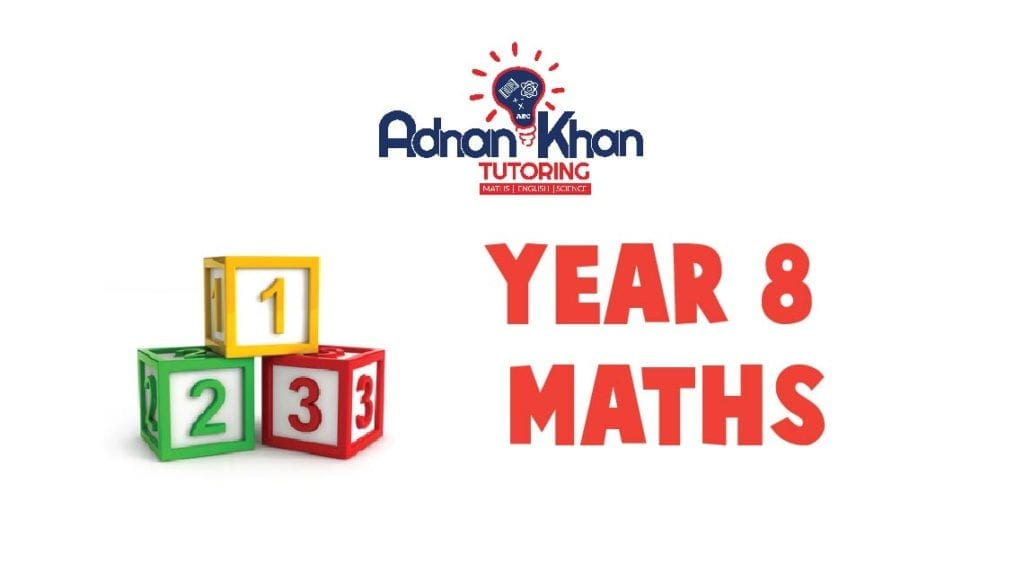# Year 8 Maths Tutors in High Wycombe

Year 8 is essential as it is crucial to building a solid foundation for the GCSE exams. Hiring a year 8 Maths tutor can help the child with their difficult Maths lessons. Math needs practice and time; the child can get them with a dedicated Maths tuition. A child cannot learn everything on their own; they need someone to help through their academics. Hiring a private tutor for year 8 in High Wycombe may not be affordable for many parents. Online Maths tuition is affordable and provides a practical learning experience with improved teaching methods.

At Adnan Khan Tutoring, we offer an exclusive range of online Year 8 Maths tuition in High Wycombe and across the UK. We provide a hi-tech tutoring platform using advanced technology and methods. Get your child exclusive year 8 Maths tutoring with Adnan Khan Tutoring. We offer affordable and feasible membership plans, monthly and yearly. We keep the parents updated with the child’s progress. You can try our free trial for two weeks as well.

## What lessons do we offer in our online year 8 maths tuition?

Our online year 8 Maths lessons are divided into four sections. The first section is Number Crunching which includes lessons Multiplying Decimals, Dividing Decimals, Special Number Sequences, HCF and LCM, Prime Numbers, Ratio, Fractions, Best Buy, Fraction Arithmetic, More Fraction Problems and other vital lessons.

The second section is Shapes and Area, including the following types of lessons.

•  Regular Polygons
•  Symmetry
•  Families of Triangle
•  Vertices, Faces and Edges
•  Perimeter and more

The third section is Shape, Space and Measures, with lessons:

•  Alternate and Corresponding Angles
•  Interior and Exterior Angles of Polygons
•  Geometric Proof
•  Geometric Properties
•  Constructions
•  Areas of a Triangle
•  Area of a Parallelogram and more

The last section is Handling Data which includes lessons related to data handling:

•  Probability
•  Probability Scales
•  Mutually Exclusive Events
•  Calculating Probabilities
•  Experimental Probability Experimental and Theoretical Probability and more lessons.

Year 8 Mathematics

Algebra

• Algebraic shorthand
• Like Terms
• Expanding Brackets and Factorising
• Expand and Simplify
• Using Algebra with Shapes
• Index Notation with Algebra
• Linear Functions
Finding Functions from Inputs and Outputs
• Graphs of Functions
• Gradient of a Straight Line
• Real-Life Graphs
• Solving Equations
• Equations Involving Negative Numbers
• Equations with Unknowns on Both Sides
• Trial and Improvement
• Substituting into Expressions and formulae
• Construction Equations

Number

• Multiplying and Dividing Negative Numbers
• HCF and LCM
• Powers and Roots
• Prime factors
• Linear Sequences
• Describing Sequences
• Solving problems
• Fractions and Decimals
• Multiplying and Dividing Decimals
• Percentages
• Percentage Increase and Decrease
• Powers of 10
• Rounding
• Estimations
• BODMAS
• Proportion
• Ratio
• Number and Measures
• Using Algebra, Graphs and Diagrams
• Logic and Proof

Shape, Space and Measures

• Alternate and Corresponding Angles
• Interior and Exterior Angles of Polygons
• Geometric Proof
• Geometric Properties
• Constructions
• Areas of a Triangle
• Area of a Parallelogram
• Volume of a Cuboid
• The Circle
• Circumference of a Circle
• Area of a Circle
• Surface Area and Volume of Prisms
• Converting Metric Units
• Imperial Units
• Congruent Shapes
• Combinations of Transformations
• Enlargements Planes of Symmetry
• Shape and Ratio
• Plans and Elevations
• Scale Drawings
• Finding the Mid-point of a Line

Handling Data

• Probability
• Probability Scales
• Mutually Exclusive Events
• Calculating Probabilities
• Experimental Probability Experimental and Theoretical Probability
• Statistical Calculations
• Statistical Surveys
• Collecting Data and Frequency Tables
• Drawing Frequency Diagrams
• Statistical Charts and Graphs
• Stem-and-Leaf Diagrams
• Interpreting Graphs and Diagrams
• Analysing Data
• Assumed Mean
• Comparing Data
• Comparing Sets of Data

## Enrol Now

#### £129.00

per month for 12 total payments
12 months of access

Categories:

Scroll to Top